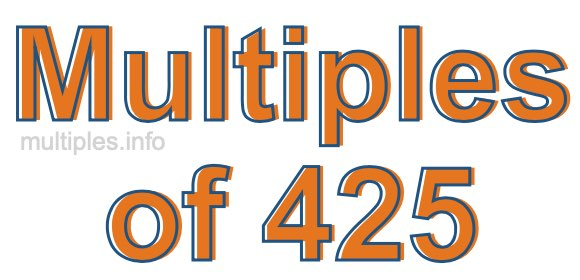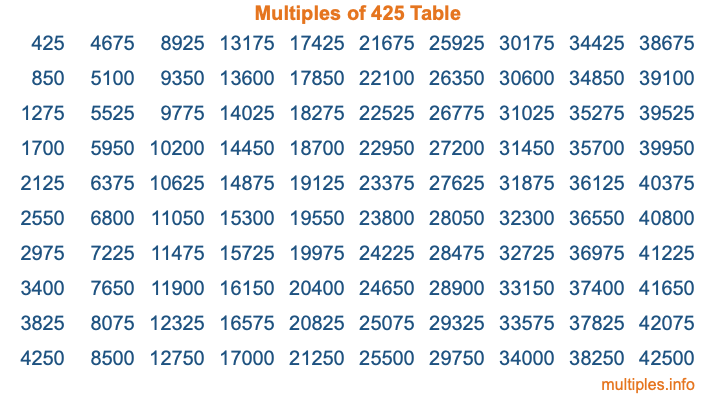Multiples of 425Welcome to the Multiples of 425 page. Here we will first teach you everything you will ever need to know about the multiples of 425, and then give you a study guide summary of everything we taught you to make sure you remember it all. Use this page to look up facts and learn information about the multiples of 425. This page will make you a multiples of four hundred twenty-five expert!

Definition of Multiples of 425
Multiples of 425 are all the numbers that when divided by 425 equal an integer. Each of the multiples of 425 are called a multiple. A multiple of 425 is created by multiplying 425 by an integer.

Therefore, to create a list of multiples of 425, you start with 1 multiplied by 425, then 2 multiplied by 425, then 3 multiplied by 425, and so on for as long as you want. Thus, the list of the first five multiples of 425 is 425, 850, 1275, 1700, and 2125. To see a larger list of multiples of 425, see the printable image of Multiples of 425 further down on this page. We also have a category where you can choose any nth multiple of 425.

Multiples of 425 Checker
The Multiples of 425 Checker below checks to see if any number of your choice is a multiple of 425. In other words, it checks to see if there is any number (integer) that when multiplied by 425 will equal your number. To do that, we divide your number by 425. If the the quotient is an integer, then your number is a multiple of 425.

Is  a multiple of 425?

Least Common Multiple of 425 and ...
A Least Common Multiple (LCM) is the lowest multiple that two or more numbers have in common. This is also called the smallest common multiple or lowest common multiple and is useful to know when you are adding our subtracting fractions. Enter one or more numbers below (425 is already entered) to find the LCM.

Check out our LCM Calculator if you need more details about the Least Common Multiple or if you need the LCM for different numbers for adding and subtraction fractions.

nth Multiple of 425
As we stated above, 425 is the first multiple of 425, 850 is the second multiple of 425, 1275 is the third multiple of 425, and so on. Enter a number below to find the nth multiple of 425.

th multiple of 425

Multiples of 425 vs Factors of 425
425 is a multiple of 425 and a factor of 425, but that is where the similarities end. All postive multiples of 425 are 425 or greater than 425. All positive factors of 425 are 425 or less than 425.

Below is the beginning list of multiples of 425 and the factors of 425 so you can compare:

Multiples of 425: 425, 850, 1275, 1700, 2125, etc.

Factors of 425: 1, 5, 17, 25, 85, 425

As you can see, the multiples of 425 are all the numbers that you can divide by 425 to get a whole number. The factors of 425, on the other hand, are all the whole numbers that you can multiply by another whole number to get 425.

It's also interesting to note that if a number (x) is a factor of 425, then 425 will also be a multiple of that number (x).

Multiples of 425 vs Divisors of 425
The divisors of 425 are all the integers that 425 can be divided by evenly. Below is a list of the divisors of 425.

Divisors of 425: 1, 5, 17, 25, 85, 425

The interesting thing to note here is that if you take any multiple of 425 and divide it by a divisor of 425, you will see that the quotient is an integer.

Multiples of 425 Table
Below is an image of the first 100 multiples of 425 in a table. The table is in chronological order, column by column. The first column has the first ten multiples of 425, the second column has the next ten multiples of 425, and so on.The Multiples of 425 Table is also referred to as the 425 Times Table or Times Table of 425. You are welcome to print out our table for your studies.

Negative Multiples of 425
Although not often discussed or needed in math, it is worth mentioning that you can make a list of negative multiples of 425 by multiplying 425 by -1, then by -2, then by -3, and so on, to get the following list of negative multiples of 425:

-425, -850, -1275, -1700, -2125, etc.

Multiples of 425 Summary
Below is a summary of important Multiples of 425 facts that we have discussed on this page. To retain the knowledge on this page, we recommend that you read through the summary and explain to yourself or a study partner why they hold true.

There are an infinite number of multiples of 425.

A multiple of 425 divided by 425 will equal a whole number.

425 divided by a factor of 425 equals a divisor of 425.

The nth multiple of 425 is n times 425.

The largest factor of 425 is equal to the first positive multiple of 425.

425 is a multiple of every factor of 425.

425 is a multiple of 425.

A multiple of 425 divided by a divisor of 425 equals an integer.

425 divided by a divisor of 425 equals a factor of 425.

Any integer times 425 will equal a multiple of 425.

Multiples of a Number
Here you can get the multiples of another number, all with the same attention to detail as we did for multiples of 425 on this page.

Multiples of
Multiples of 426
Did you find our page about multiples of four hundred twenty-five educational? Do you want more knowledge? Check out the multiples of the next number on our list!